# Shedding Light on Motion Episode 7: Newton’s Second Law

In Episode 7, Newton’s Second Law, we answer the most important question that has ever been asked: how does a magician pull a tablecloth out from under a dinner set? No, seriously, we look at the so simple and yet so powerful equation Fnet = ma. Newton’s Second Law tells us how to calculate the amount of force required to accelerate something by a given amount.

The Shedding Light on Motion series is a visual treasure trove of demonstrations, animations, and explanations of all things motion! To an extent we’re all familiar with motion because we all move and we see movement everywhere, but a detailed knowledge of motion has allowed us to build the wonderful modern world that we live in.

The trailer below contains a 4-minute excerpt from the program.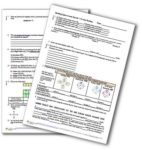The Episode 7 Question Sheet for Students:QS7 Newton’s Second Law.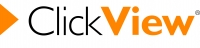If you have ClickView, watch the whole episode here.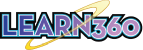If you have Learn360, watch the whole episode here.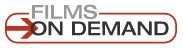If you have Films on Demand, watch the whole episode here.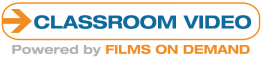If you have Classroom Video, watch the whole episode here.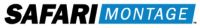Most of our videos are also available on SAFARI Montage. Just log in and do a quick search.Don’t have any of the above? Rent or buy the Shedding Light series and/or individual programs from our Vimeo page!!

The Transcript (which can be used as a textbook)

Contents:
Part A: Introduction.
Part B: Fnet = ma. Newton’s First Law tells us that things won’t accelerate unless a force acts on them. Newton’s Second Law tells us how much force is required to accelerate something by a given amount. It’s such a simple yet such a powerful equation!
Part C: Friction and Mass. More massive objects require more force to accelerate by a given amount than less massive objects. In Part C, we clear up one of the biggest misconceptions in Science. We also explain how a magician can pull a tablecloth out from under a dinner set.
Part D: Newton’s Second Law of Car Motion. How can cars accelerate faster than trucks when they have much smaller engines than trucks? And what exactly is a crumple zone?
Part E: Free Fall Acceleration. Why do all objects in free fall accelerate at a rate of 9.8 m/s/s? There’s a surprisingly simple explanation.
Part F: Mass and Weight. If you want to lose weight, you can go to the moon or to the International Space Station, but unfortunately you will still have the same mass.

Part A: Introduction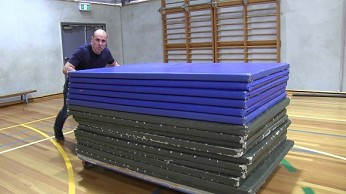In this program, we’re going to look at Newton’s Second Law of Motion. It follows on, naturally enough, from Newton’s First Law of Motion, which states that an object will remain at rest or move with a constant velocity unless an unbalanced force acts on it. In other words, nothing’s ever going to speed up or slow down or change direction unless a force acts on it. Newton’s Second Law of Motion gets a little more mathematical than his First Law of Motion and it introduces an equation.

So Newton’s First Law of Motion says that a force is needed to accelerate something, but Newton’s Second Law of Motion actually allows us to calculate how big a force has to be to accelerate an object by a given amount. Let’s take a look at it.

Part B: Fnet = ma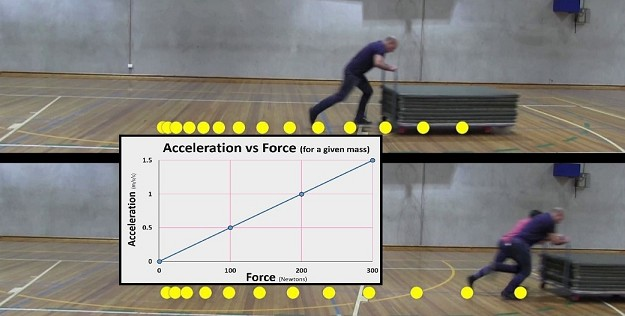We know that in order to accelerate something a force needs to be applied to it. It turns out that force and acceleration are directly proportional to each other. If I apply a force to this trolley it will accelerate by a certain amount. If I keep the mass of the trolley the same, but I increase the force, the trolley accelerates at a greater rate. A greater force causes an object to speed up faster than a smaller force does.

A graph of acceleration vs force (for a constant mass) might look something like this. The more force, the more acceleration. We can say that the acceleration is directly proportional to the force. If you double the force for example, the object’s acceleration doubles. If you increase the force by 20%, the object’s acceleration will increase by 20%.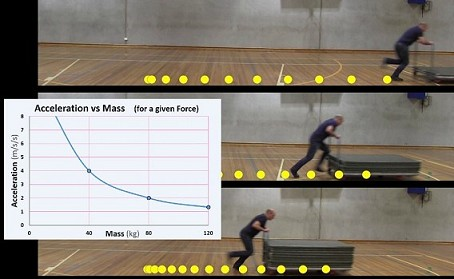Now what happens if we keep the force the same, but vary the mass? When the trolley has only 1 green mat on it, I can accelerate it fairly quickly. If I increase its mass, and then apply the same force, the acceleration is a lot smaller. Increasing the mass again results in an even smaller acceleration. It’s quite clear that for any given force, a larger mass will accelerate at a lower rate than a smaller mass. This graph shows the relationship. As the mass gets bigger, the acceleration gets smaller. This is called an inverse relationship.

We can express the relationship between force, mass and acceleration in a single equation: F = ma. In this equation, m = the mass in kilograms (kg), a = the acceleration in metres/second/second (m/s/s or m/s2), and F = the force that acts on the object in a unit that you might not hear every day called the newton (which is given the symbol N). The newton is named after Isaac Newton of course. The equation F = ma is Newton’s Second Law.

So let’s do an example so that you can see how the equation works and so that you can get an understanding of what a Newton is.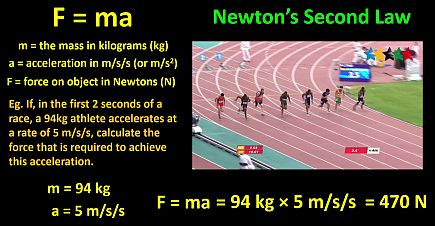If, in the first two seconds of a race, a 94kg athlete accelerates at a rate of 5 m/s/s, calculate the force that is required to achieve this acceleration. Well, m = 94 kg, a = 5 m/s/s and so the force, F, = ma which equals 94 kg x 5 m/s/s which equals 470 Newtons.

Let’s do another example. Calculate the force required to accelerate a 900 kg car from 0 to 60 km/hr in 10 seconds. Well we know that F = ma. m = 900 kg, but what is a? The acceleration, a = the change in velocity over the change in time. 60 km/hr = 16.7 m/s (remember you always have to be careful about what units you use). So the acceleration, a = the change in velocity over the time taken (16.7 – 0 m/s/s over 10 seconds) which equals 1.67 m/s/s. The force (on the car) was therefore 900 kg x 1.67 m/s/s which is about 1500 Newtons (1503 N).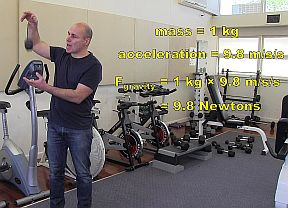Let’s look at one more example. What’s the force of gravity on this 1 kg weight when it’s in free fall? Well, its mass is 1 kg and its acceleration is 9.8 m/s/s, so the force of gravity acting on it is 1 x 9.8 which equals 9.8 N. This is actually a convenient way of visualizing the unit of the newton. A 1kg mass experiences just under 10 Newtons of force due to gravity.

Of course the equation can be re-arranged to make “a” the subject, a = F/m or to make “m” the subject, m = F/a.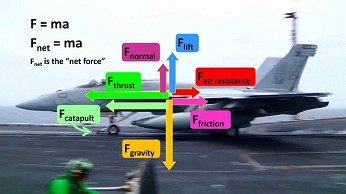Now we’ve seen how at any given moment there are usually many different forces acting on an object. There’s thrust, for example, air resistance, friction, gravity, lift, the normal force and other forces such as in this case, the force of the catapult that helps push the plane as it takes off.

So when we say that F = ma, the F (for force) is actually the sum of all the forces acting on the object at that moment. The equation is therefore often written as Fnet = ma, where Fnet is the net force, or in other words the sum of all the forces acting on the object. Let’s look at a simple example of what I’m talking about.Two people are pushing a car, one with a force of 100 N and the other with a force of 150 N. Assuming that there is no friction, just to keep things simple, what is the net force on the car? Well, quite simply, since both of us are pushing in the same direction, the net force is 100 N + 150 N which is 250 N. Pretty simple.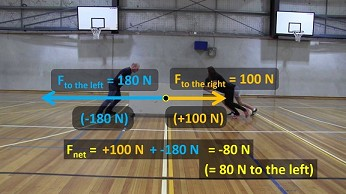Now we’ve seen in previous episodes that velocity is the speed of an object and the direction that it’s travelling in. Your velocity can be to the right, negative, upwards, to the North or whatever. Forces also have a direction. When you add forces you have to take their direction into account. If, on this bench, I apply a force of 100 N to the right, I’ll call it +100N, but two others apply a combined force of 180 N to the left (I’ll call it -180N), the net force is +100 N plus -180 N which equals -80 N, or 80 N to the left.

Let’s do another example. A 50 kg bike rider is on a 15 kg bike. If she’s peddling with a thrust of 100 N to the left which I’ll use as my positive direction in this example, but the forces of friction and air resistance which I’ll represent with a single arrow and call them the resistance forces, add up to 70 N to the right, what’s the net force acting on the rider and her bike and what’s her acceleration?The net force equals +100 N + -70 N (since the resistance forces are acting in the opposite direction to the cyclist’s direction). This equals +30 N, or 30 N to the left, the direction that the cyclist is facing. The mass of the rider and the mass of the bike add up to 65 kg. Since Fnet = ma, a = Fnet/m. Her acceleration is therefore 30 N/65 kg which is about 0.46 m/s/s. Here of course we’ve ignored the force of gravity and the normal force. Since they are equal in size but act in opposite directions, they balance out and therefore don’t affect the rider’s acceleration in any way.

Now the Fnet = ma equation tells us that the direction of the net force is also the direction that the object will accelerate in. Since this jet is accelerating towards our left, then the net force acting on it must also be towards our left. Even though there are lots of different forces acting, the overall force on the jet, that is, the “net force”, can be represented by a single arrow.

And just repeating the equation, if you know the mass of an object, and the acceleration of the object, it’s very easy to calculate the net force acting on an object: Fnet (on object) = mobject x aobject

Now, every plane model that has ever flown has to have had a first flight. This is the Airbus company’s A380, the largest passenger plane in the world, taking off for its first ever flight. How did the engineers know that the plane was going to take off? You can’t just build a plane and hope that it will fly. You have to design it first, test the thrust that the engines will produce, and use models to calculate the force of air resistance and the lift that the wings will generate. At every step of the way, there’s a whole lot of complex mathematics but also, in amongst it all, is the simple equation Fnet = ma.

Without the equation F = ma, we just wouldn’t be able to fly fly away!!Now when pushing a trolley, it’s hard to initially accelerate it, but once you get up to speed, it’s relatively easy. Why is that? Well, when you first start pushing, you need to apply enough force to accelerate the object and to overcome friction. However, once you get to the constant velocity that you want, the net force required is zero. The forward force simply needs to balance the force of friction, which isn’t really that great. So it becomes much easier.Let’s look at the numbers. The mass of the trolley is 100 kg and it accelerated at a rate of 1 m/s/s. We can therefore calculate that the net force acting on it initially was about 100 N. The frictional force was about 30 N so I had to apply a thrust force of 130 N onto it to provide a net force of 100 N. The net force = the force of thrust plus the force of friction so 130 N to the right (let’s call it positive 130 N) plus 30 N to the left (let’s call it negative 30 N) = a net force of 100 N to the right.

However, once I got to a comfortable walking speed, to maintain that constant velocity, I only needed to apply a force of 30 N to the right to exactly match the 30 N force of friction to the left. To accelerate the trolley again, I have to increase the forward force on it over and above the force of friction.

So, if Fnet = ma and if the acceleration = 0, then the net force (Fnet) must also be zero. Anything times zero is zero. This is actually just what Newton’s First Law states: an object will remain at rest or move with a constant velocity unless an unbalanced force acts on it. If the net force on an object is zero, in other words there’s no unbalanced force acting on it, its acceleration will be zero, and so it’s not going to speed up or slow down. Newton’s First Law is actually just a special case of Newton’s Second Law!

Part C: Friction and Mass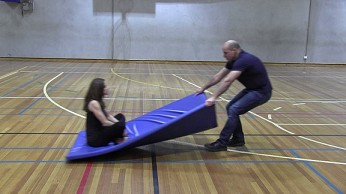Now sometimes people get confused about mass and friction. They think that it’s harder to accelerate a more massive object because there’s more friction. To a small extent that’s true. The friction between the blue mat and the floor is quite small, but it increases if a weight is placed onto the mat. However, when wheels are involved, the increase in friction is usually quite small.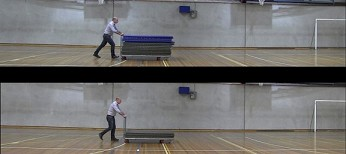Pushing these two trolleys once I’ve got up to a comfortable walking speed requires more or less the same force because I only have to apply enough force to overcome friction, which is more or less the same in both cases because of the wheels.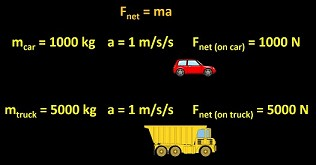So, though changes in friction may be a factor, the main reason that it’s harder to accelerate a more massive object is that, well, more mass simply needs more force to accelerate it by any given amount. If you have two trolleys with different masses, but you want them to accelerate at the same rate, you need to apply a larger force on the more massive one. If you want to accelerate a 1000 kg car by 1 m/s/s, you need 1000 N. If you want to accelerate a 5000 kg truck by 1 m/s/s, you need 5000 N.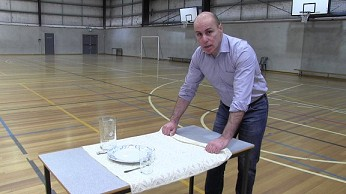So now let’s get to the questions I posed in our last program. Why is it that when the trolley accelerates slowly, the ball accelerates with the trolley, but when the trolley accelerates quickly the ball doesn’t keep up and, from the trolley’s frame of reference, actually goes backwards. Likewise why does the dinner set move with the tablecloth when it’s pulled slowly but not when it’s pulled quickly.

The force that allows the dinner set to initially accelerate with the table cloth comes from friction, which is fairly small. Though we usually think of friction as a force that slows things down, it’s also the force that stops things from slipping, so in this case, friction is responsible for applying a force on the plate towards the right. However, as I said, the frictional force is very small. This small force can accelerate the pieces of the dinner set only at a very low rate. Small force, small acceleration. If the tablecloth is pulled quickly, the force of friction is nowhere near big enough to accelerate the dinner set pieces at the same rate as the tablecloth. They only accelerate by a tiny amount in the time that the small force of friction acts and so by the time the tablecloth is no longer in contact with them, they’ve barely moved.I can actually measure the force of friction involved, for example on the plate, by using this spring balance. The spring balance, which is marked in Newtons, reads about 2.2 Newtons as I drag the plate with a constant velocity across the tablecloth. Remember, at a constant velocity, the forward force is exactly equal in size to the force of friction, so the force of friction must also be about 2.2 Newtons.It’s this frictional force of 2.2 Newtons that acts on the plate when I pull the tablecloth. Now the plate has a mass of about 700 grams: 0.7 kilograms. Using the Fnet = ma equation, we can calculate that the maximum acceleration that a 0.7 kg plate can undergo if a force of 2.2 Newtons is applied to it is only 3.1 m/s/s. However, here, the tablecloth accelerated at a rate of about 30 m/s/s.

The frictional force on the plate just isn’t big enough to make it accelerate as quickly as the tablecloth does. Combining this with the fact that the small force acts for such a small amount of time we end up with a plate that barely moves.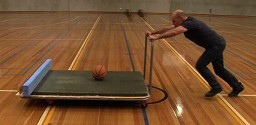It’s the same situation here. I apply a force to the trolley, which makes it accelerate, but the force making the ball accelerate comes only from the friction between the ball and the trolley. And this force is tiny. As a result, the ball can accelerate slowly when the trolley accelerates slowly, but it can’t accelerate quickly, when the trolley accelerates quickly, because the frictional force on the ball is just too small. From the trolley’s frame of reference therefore it seems to roll backwards, although from the Earth’s frame of reference it just kind of gets left behind as the trolley moves underneath it.

Part D: Newton’s Second Law of Car MotionMotorbikes can typically accelerate at a much higher rate than cars, and cars can typically accelerate at a much higher rate than trucks. The acceleration of a vehicle is determined by the ratio of the force that it can generate to its mass. In other words, a = F/m. A truck typically has a much larger and much more powerful engine than a car, but because the mass of a truck is so great, it usually has a smaller acceleration than a car.For example, if a 5000 kg truck has an engine that can provide 4000 N of force, it can accelerate at 0.8 m/s/s, which is about 2.9 km/hr per second. If a 1000 kg car has an engine that can provide a much smaller force of 1500 N, it can accelerate at a much higher rate of 1.5 m/s/s, which is about 5.4 km/hr every second. Obviously the numbers vary a lot from vehicle to vehicle.

Bikes used by professional cyclists are made of really lightweight materials because having less mass means that the cyclists can accelerate at a higher rate.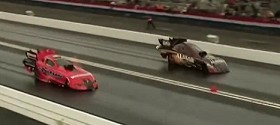Drag-racing cars can accelerate at a huge rate because they have really powerful engines which can provide a huge force, and they have very little mass. They don’t have brakes, a radiator, back seats, air conditioning units or anything like that and their external panels aren’t made of steel but rather lightweight materials such as carbon fibre.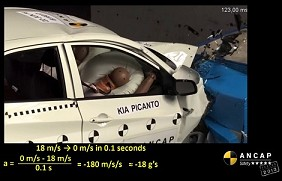Now as great as a drag car’s acceleration is, it’s nowhere near as great as the deceleration of a car that undergoes a collision. In this crash, the dummy passengers slowed from 18 m/s (64 km/hr) to 0 in only about 0.10 seconds, which is an acceleration of about -180 m/s/s (or about -18 g’s). The force required to stop the car and its dummy passengers was huge but if the passengers were human they probably would have survived, although they may still have been injured of course. Cars are designed to slow down as slowly as possible in a collision. The smaller the deceleration, the smaller the force.

By the way, by international agreement, crash tests like these ones are all done at 64 km/hr so that the effectiveness of the safety features can be compared fairly.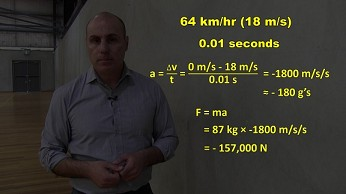Let’s actually look at some numbers. If I was somehow moving at 64 km/hr (18 m/s) without any kind of protection and crashed into a solid concrete wall, (SLAP) I would come to a complete stop in only about 0.01 seconds. My acceleration would be the change in velocity (that is, my final velocity minus my initial velocity) over the time taken, which is about -1800 m/s/s ((0 – 18)/0.01) or about -180 g’s. According to the F = ma formula, the force exerted on my 87 kg body would be a huge 157,000 N. The negative sign indicates that the force acts in the opposite direction to the direction that I was travelling in.

Assuming my skull actually remained intact, which I doubt, my brain would come forward and squash up against my skull. The connective tissue that holds my brain in place is just nowhere near strong enough to withstand the forces involved in a collision at 64 km/hr that lasts only 0.01 seconds. I can simulate the brain moving forward in a collision using this skull and a balloon. I can also represent a more severe collision and ask you to use your imagination!However if it was me in this collision, and I came to a stop from an initial speed of 64 km/hr in a time of 0.1 seconds instead of 0.01 seconds, my deceleration would be, as we saw earlier, a relatively small -180 m/s/s. The force acting on me would only be about 16,000 N, which is still a lot, but most people would survive this accident.

Cars actually have a number of safety features that are designed to slow us down slowly in a collision and so to reduce the forces acting on us.

Firstly cars are built with two what are called “crumple zones”; one at the front and one at the back. Steel beams behind the external panels crumple inwards during a collision and increase the time that it takes the car to come to a stop. If you stop really quickly, the force has to be really large, but by increasing the time it takes to stop the forces are reduced. It’s fairly obvious which car here is safer!

The second major safety feature is the seat belt which holds you in place in the car so that you slow down at about the same rate as the car does. Thirdly, the air bag also slows you down more slowly. Seatbelts and airbags also spread the stopping force over a wide part of the body which of course decreases the force on any given part of the body.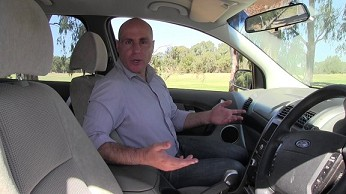Without an airbag, a seatbelt, and a well-designed crumple zone, the front occupants in a car would keep moving forward in a collision until their head hits the windscreen, which, similar to crashing into a concrete wall, would exert a huge force that all too often would result in a lethal injury.

Ideally of course you would never have an accident and you would always decelerate really slowly just by braking. In normal braking, the forces are obviously safe.

Many cars actually have collision avoidance systems built in. On-board radars can detect obstacles and automatically apply the brakes if the car’s computer determines that a collision will occur if it doesn’t act. Given that most accidents are caused by driver error, and that many of these accidents involve a distracted driver, these kinds of systems can be real life savers.

Part E: Free Fall Acceleration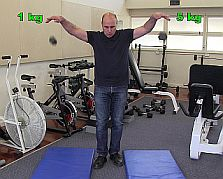We saw in the Acceleration program that all objects, regardless of their mass, accelerate at the same rate when they’re in free fall: 9.8 m/s/s (or 9.8 m/s2 if you prefer), assuming that air resistance is very small. Now why is that? Let’s consider this 1kg mass. We’ve seen that when it’s in free fall, the force of the Earth’s gravity is pulling it downwards. We know its mass is 1 kg and we know that its acceleration is 9.8 m/s/s. Applying the formula Fnet = ma, we can work out that the force of gravity on it is 9.8 Newtons.

But the same force of gravity must be acting on it right now, only now it’s not falling because I’m providing an upwards force of 9.8 N which is counteracting the downwards force of gravity. So whether the 1 kg mass is falling or stationary there is always a gravitational force of 9.8 Newtons on it. In fact because of gravity there is a force of 9.8 N on every kilogram of mass on the Earth’s surface. To express this concept that every kilogram of mass experiences 9.8 Newtons of force because of gravity (well, on the Earth’s surface that is) scientists write g = 9.8 Newtons/kilogram.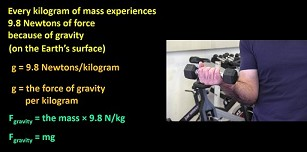We saw in our Acceleration program that 1 g = 9.8 m/s/s, the acceleration due to gravity but scientists use the same symbol, g, for the force of gravity per kilogram of an object. The same symbol is used because the two concepts are related. Gravity causes objects to accelerate.

So we can calculate the force of gravity on any object with a simple equation:

Fgravity = the mass × 9.8 N/kg, or Fgravity = mg

The force of gravity on a 2 kg mass (which you can think of as 2 1kg masses, I suppose) is 2 kg x 9.8 N/kg which equals 19.6 Newtons. To hold up a 2 kg mass, I obviously need to provide a greater upwards force than what I need to hold up a 1 kg mass. The force of gravity pulling down on a 5 kg mass is (on screen: 5kg x 9.8 N/kg) 49 Newtons. I therefore have to provide an upwards force of 49 newtons to balance the downwards pull of gravity on it. The force of gravity pulling down on 40 kg of mass is just under 400 Newtons. My mass is about 87 kg and so the Earth is applying a downwards force on me of about 870 Newtons.Of course this equation only applies to objects on or near the Earth’s surface. On the surface of the moon, the force of gravity per kilogram is different. In fact it’s only 1/6 of what it is on Earth.

Now why do different masses in free fall all accelerate at the same rate of 9.8 m/s/s (assuming that air resistance is very small)? Let’s allow the 3 weights to fall but then freeze frame them. The force of gravity on a 1 kg mass is 9.8 N. Assuming there’s no air resistance, this is the only force acting on it and therefore it’s the net force acting on it. Now Fnet = ma so a = Fnet/m which in this case is 9.8/1 since m = 1 kg. The acceleration must therefore be equal to 9.8 m/s/s. The force of gravity on the 2 kg mass is 2 x 9.8 which equals 19.6 N. This force is acting on a 2 kg mass, so the acceleration of the 2 kg mass is the same as that of the 1 kg mass: 9.8 m/s/s. A 5 kg mass experiences a force of 5 x 9.8 or 49 N. Applying Newton’s formula we get 49 N / 5 kg which equals, once again, 9.8 m/s/s.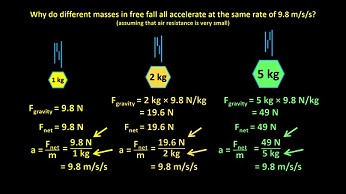So, all objects in free fall accelerate at the same rate. More massive objects experience an overall greater force, but this force is applied over a larger mass, so the acceleration ends up being the same.

I can actually demonstrate this using real weights. The 1, 2, and 5 kg masses all accelerated at the same rate: 9.8 m/s/s. It’s like what we saw earlier. If you double the force on double the mass it results in the same acceleration.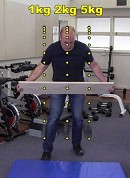Purely conceptually, if two 1 kg mass accelerate at 9.8 m/s/s when they’re in free fall, why should sticky taping them, or welding them or gluing them or whatever together make any difference? This combined mass is really made of individual masses, so of course as a 2-kg mass it’s going to accelerate at the same rate as a 1-kg mass.

However, this only applies when air resistance is negligible. We’ve assumed so far that the force of gravity is the net force since the force of gravity is the only force acting. What happens though when air resistance is significant, which is a lot of the time of course?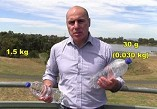Let’s see what happens when I drop these two bottles which have the same shape but which have very different masses. This one has a mass of about 1.3 kilograms because it’s full of water, whereas this one has a mass of only about 30 grams. Clearly the heavier bottle accelerated at a greater rate than the lighter bottle. Why is that? How does air resistance affect the acceleration of different objects?

Well, let’s look at 3 different masses of mass 1, 2, and 3 kilograms. This time the masses will have the same shape and dimensions which means that they have the same air resistance at any given speed, just like our two bottles.

The force of gravity on each one is 9.8 N, 19.6 N, and 29.4 N. If all three start falling, the initial air resistance is basically zero, so as we’ve seen the force of gravity will be the only force acting on them. They all therefore start to accelerate at 9.8 m/s/s. Let me freeze frame them again after 1 second when they reach a speed of about 9.8 metres/second. By now, air resistance actually becomes a factor.

Let’s now assume that the air resistance at this speed on all three masses is now 4 Newtons. It’s not as if the air resistance suddenly jumps up from 0 to 4 Newtons, it actually increases as an object gets faster and faster, but I just want to keep it simple. The net force on each mass is now the force of gravity plus the force of air resistance.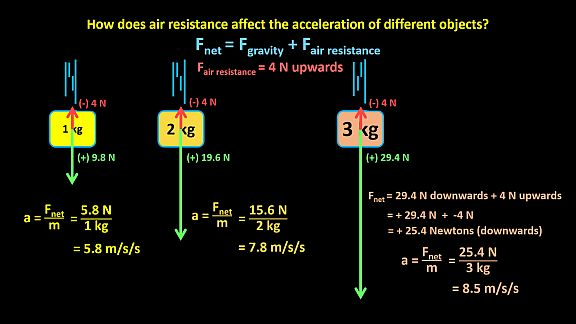The 1-kg mass is now experiencing a net force of 9.8 N of gravity downwards + 4 N of air resistance upwards which is 5.8 N downwards. Here I’ve taken the downwards direction as positive. Using the a = Fnet / m formula, we find that its acceleration is therefore now only 5.8 m/s/s.

The 2-kg mass is now experiencing a net force of 19.6 N of gravity downwards + 4 N of air resistance upwards which equals 15.6 N downwards. As a percentage, the force of air resistance is smaller on the 2-kg mass than it is on the 1-kg mass. The acceleration of the 2-kg mass is (a = Fnet / m) only 7.8 m/s2. Less than its initial acceleration, but more than the acceleration of the 1-kg mass. If we repeat the procedure with the 3 kg mass, we find that its acceleration after half a second is now 8.5 m/s2.

As a result of air resistance, the three masses are no longer accelerating at the same rate. The 3-kg mass will therefore end up falling faster than the 1-kg mass.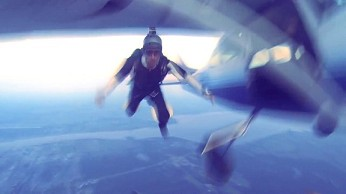Since air resistance increases as the velocity of an object increases, as falling objects continue to accelerate downwards they may eventually, if they fall fast enough, reach a speed where air resistance becomes equal to the force of gravity. At that point, the net force is zero, since the size of the downwards force equals the size of the upwards force, and so the acceleration is zero: they will just keep moving at the same velocity, without undergoing any more acceleration. This velocity is called the “terminal velocity”.A graph of velocity vs time for an object in free fall looks something like this. The velocity increases but it kind of levels off after a little while. I haven’t put any numbers on the graph because the terminal velocity varies depending on (a) the mass of the object which affects the force of gravity on it and on (b) the size of the air resistance. The terminal velocity of a skydiver is about 200 km/hr depending on their mass and on how they orientate their body, but the terminal velocity of a sky diver who has their parachute deployed is only about 5 km/hr.

The terminal velocity of the 3 kg mass will be greater than the terminal velocity of the 1-kg mass because it has to reach a greater velocity before the air resistance matches the force of gravity on it.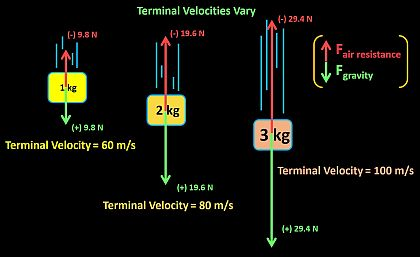So once, again, if (or when) air resistance is small, the acceleration of all objects in free fall is the same: 9.8 m/s/s. Even over a 10 metre drop, the 700 gram basketball and I with a mass 87 kg accelerated at the same rate. If (or when) air resistance is relatively large compared to the force of gravity, lighter objects don’t accelerate as quickly.

Part F: Mass and Weight

We’ve seen that the force of gravity on this 1 kg dumbbell is 9.8 N and that the force of gravity on this 2 kg dumbbell is 19.6 N. In everyday language we often say things like this dumbbell weighs 1 kg and this dumbbell weighs 2 kg. But mass and weight are not really the same thing, even though we often use the words interchangeably.You’ll sometimes see, for example, that food packaging is labelled with the net weight of the contents in grams or kilograms, but most labelling just indicates the number of grams without using the word “weight”. In science, the mass of an object is the amount of matter in the object and typically it’s measured in kilograms. An object’s mass is a measure of how difficult it is to accelerate it according to the equation F = ma. The weight of an object is actually the force of gravity pulling the object downwards and it’s measured in Newtons.

Scientifically speaking, the mass of the 1 kg dumbbell is 1 kg but its weight, which is the force of gravity on it is 9.8 N. The mass of the 2 kg dumbbell is 2 kg but its weight is 19.6 N.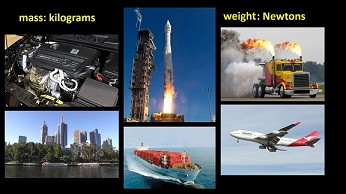Now I don’t want to make a big deal of it, because, as I said in everyday language we never quote an object’s weight in Newtons. We would typically say something like “I weigh 87 kilograms”. However when scientists and engineers are designing and constructing engines, rockets, trucks, buildings, ships, planes, and a whole lot of other things, they have to be specific, so they often quote an object’s mass in kilograms and its weight, that is, the force of gravity on it, in Newtons. The concrete and steel that supports a building for example has to be strong enough to provide an upwards force that will withstand the downwards force of gravity acting on it.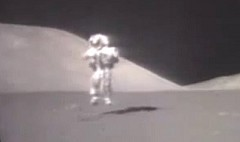Your mass doesn’t depend on where you are: if you have a mass of 70 kilograms on Earth, here we can see Neil Armstrong and Buzz Aldrin suiting up for their historic moon landing in 1969, then you would have the same mass on the moon. However your weight does change depending on the force of gravity that you feel. The gravitational pull of the moon is only about 1/6 of what it is on Earth so if you were on the moon, your mass would be the same as it is on Earth, but your weight would only be about 1/6 of what is is on Earth. All the equipment that the 12 astronauts carried around during the six moon landings in the late 1960s and early 1970s had the same mass as it had on Earth, but it was easier to lift and to carry around on the moon because it was experiencing less gravity.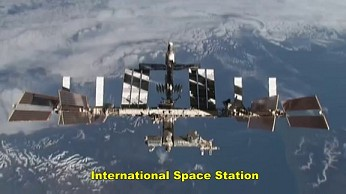In the 100m long International Space Station, orbiting about 400 km above the Earth’s surface, the astronauts and other equipment appear weightless, but they still have the same mass as what they do on Earth.

Since F = ma, if you want to accelerate something on the ISS, you still have to apply a force on it, because it’s still got mass. An object with more mass needs more force to accelerate it by a given amount than an object with less mass. Just because it floats around and gravity doesn’t pull it towards the floor, doesn’t mean that it doesn’t take a force to accelerate it. It does take a force and the force is determined by the formula F=ma.

Now here on Earth, I can’t switch off gravity, but I can kind of simulate weightlessness by suspending things from the roof. If I wanted to throw this 700g basketball on the International Space Station, I would have to apply a very similar force to the force that I need to throw it here on Earth. The only difference is that I wouldn’t have to hold it up as such against the downwards force of gravity. If I threw a 5kg medicine ball in a weightless environment, it wouldn’t accelerate as quickly as the basketball because it has more mass than a basketball. Gravity has got nothing to do with it.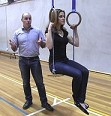If I tried to throw a 53 kg woman in a weightless environment, then I wouldn’t have to pick her up, because she would just be floating there, but I wouldn’t be able to throw her as fast as what I could throw a basketball because, even though she’s weightless, she still has mass, 53 kg of mass to be precise. And it also takes a lot more force to decelerate a larger mass of course. The equation a = F/m says that the same force acting on a larger mass produces a smaller acceleration. Here, the 5 kg medicine ball obviously accelerated at a much higher rate than the 53 kg woman did, even though I pushed both of them with the same force, the maximum force that my muscles could generate.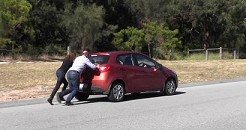This car is accelerating really slowly as it gets pushed, because it has quite a large mass. If the same car was floating in space and we applied a similar force on it to the force we’re applying on it here, it would accelerate at more or less the same rate as it’s accelerating here, although just to be accurate, here friction is involved as well.

So, mass is the amount of matter in an object and is really a measure of how difficult it is to accelerate it, whereas weight is the force of gravity pulling something downwards.

Now here on Earth, I’m able to push this pretend weightless object because I push off the floor and there’s friction between my shoes and the floor. In space though, if I was trying to accelerate a fellow astronaut that was just floating there, and I was floating as well, then as I push my colleague forwards, I would go backwards. This is because of Newton’s Third Law which we’ll look at in our next episode. See you then.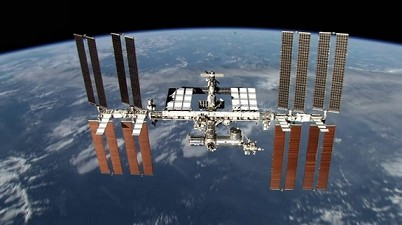All images of spacecraft, the ISS, rockets, the moon landings etc. are produced by NASA and other space agencies.Airbus footage © Airbus/master films. Used with Permission. http://www.airbus.com/broadcastroom/